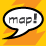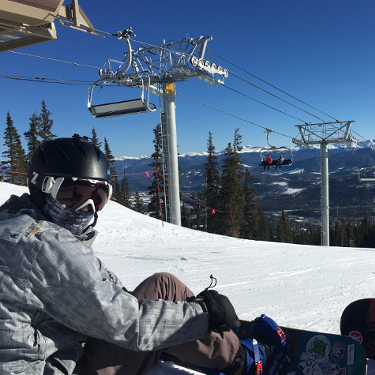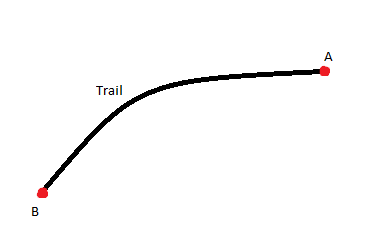# Get Attributes of Point Feature at Start and End Coordinates of Polyline

2101
6
10-27-2016 03:07 PMNew Contributor III

I have a trail network. In this trail network, each polyline segment (identified by a unique ID) is connected by an "intersection" (which is a point feature located at the junction of two trail network segments). Each trail segment has an intersection at its start and end. Each intersection is uniquely named.

What I would like to do is add the names of the intersections located at the start and end of each trail segment into the attributes of each line segment.

Without a script, the method I use:

1. Get start and end lat/longs for each trail segment
2. Create separate point features for the start and end locations with the lat/longs (and unique trail segment ID preserved)
3. Spatial join the start and end lat/longs (separately) to the intersections
4. Do a table join of the original trail segments to both the start and end locations (that have been spatially joined to the intersections) to grab the intersections for each unique name at each intersection that matched the start and end lat/longs

Overall obviously this process is clunky. So, I attempted to convert this to a script using ArcPy but am strugling with how to match the lat/longs between the point features and the trail segment start and end points. Since I have these new lat and longs in my script – is it possible to simply do a spatial join using these lat/longs without having to place them into the temporary point feature class? Or is that over-complicating an even more simple process?

Don't really need any code-specific help, just curious conceptually if what I am doing makes sense or could be performed using a better method.

Tags (6)
1 Solution

Accepted SolutionsbyMVP Honored Contributor

I think you can condense most of your steps by reading all the intersection points into a dictionary (with key = a combination of the lat/long coordinates, value = intersection name) and using a da.UpdateCursor to read the line geometries' firstPoint and lastPoint to find and populate the line table with values from the dictionary:

``````fc = 'trails' # trail FC
fc_sr = arcpy.Describe(fc).spatialReference # trail FC CRS
pts = 'intersections' # intersection feature class
pt_sr = arcpy.Describe(pts).spatialReference # intersection FC CRS
pt_attribute = 'FID' # the field to transfer
# make dictionary where {int(x)int(y):attribute}
pt_dict = {str(int(row.centroid.X))+str(int(row.centroid.Y)):row for row in arcpy.da.SearchCursor(pts, ['SHAPE@',pt_attribute], spatial_reference=pt_sr)}
with arcpy.da.UpdateCursor(fc,['SHAPE@','START','END'], spatial_reference=fc_sr) as cursor:
for row in cursor: # loop through trails
fp = row.projectAs(pt_sr).firstPoint # get firstPoint in coordinates same as points
lp = row.projectAs(pt_sr).lastPoint # get lastPoint in coordinates same as points
row = pt_dict[str(int(fp.X))+str(int(fp.Y))] # find matching intersection to firstPoint and return value
row = pt_dict[str(int(lp.X))+str(int(lp.Y))] # find matching intersection to lastPoint and return value
cursor.updateRow(row) # update values‍‍‍‍‍‍‍‍‍‍‍‍‍‍‍‍‍‍‍‍‍‍‍‍‍‍‍‍‍‍‍‍‍‍‍‍‍‍‍‍‍‍‍‍‍‍‍‍‍‍‍‍‍‍‍‍``````

edit: the above works nicely with projected coordinates because, depending on your data, you're not likely to have multiple points in the same 1m x 1m (or 1' x 1') square. You are much more likely to have multiple points in the same 1 degree x 1 degree square, so for lat/long, you would have to either project, or do some work to multiply the coordinates by some factor (e.g. 1 million) to make unique coordinate keys.

6 RepliesbyMVP Regular Contributor

So, you have something like this?And you need an attribute of point A and point B to be copied to the Trail polyline?New Contributor III

Yes, that is exactly correct.byMVP Regular Contributor

Do the point features and the line features share any uniqueID? Or are the only spatially related?New Contributor III

only spatially related.byMVP Honored Contributor

I think you can condense most of your steps by reading all the intersection points into a dictionary (with key = a combination of the lat/long coordinates, value = intersection name) and using a da.UpdateCursor to read the line geometries' firstPoint and lastPoint to find and populate the line table with values from the dictionary:

``````fc = 'trails' # trail FC
fc_sr = arcpy.Describe(fc).spatialReference # trail FC CRS
pts = 'intersections' # intersection feature class
pt_sr = arcpy.Describe(pts).spatialReference # intersection FC CRS
pt_attribute = 'FID' # the field to transfer
# make dictionary where {int(x)int(y):attribute}
pt_dict = {str(int(row.centroid.X))+str(int(row.centroid.Y)):row for row in arcpy.da.SearchCursor(pts, ['SHAPE@',pt_attribute], spatial_reference=pt_sr)}
with arcpy.da.UpdateCursor(fc,['SHAPE@','START','END'], spatial_reference=fc_sr) as cursor:
for row in cursor: # loop through trails
fp = row.projectAs(pt_sr).firstPoint # get firstPoint in coordinates same as points
lp = row.projectAs(pt_sr).lastPoint # get lastPoint in coordinates same as points
row = pt_dict[str(int(fp.X))+str(int(fp.Y))] # find matching intersection to firstPoint and return value
row = pt_dict[str(int(lp.X))+str(int(lp.Y))] # find matching intersection to lastPoint and return value
cursor.updateRow(row) # update values‍‍‍‍‍‍‍‍‍‍‍‍‍‍‍‍‍‍‍‍‍‍‍‍‍‍‍‍‍‍‍‍‍‍‍‍‍‍‍‍‍‍‍‍‍‍‍‍‍‍‍‍‍‍‍‍``````

edit: the above works nicely with projected coordinates because, depending on your data, you're not likely to have multiple points in the same 1m x 1m (or 1' x 1') square. You are much more likely to have multiple points in the same 1 degree x 1 degree square, so for lat/long, you would have to either project, or do some work to multiply the coordinates by some factor (e.g. 1 million) to make unique coordinate keys.New Contributor III

This is perfect, thanks very much for assisting an arcpy novice.